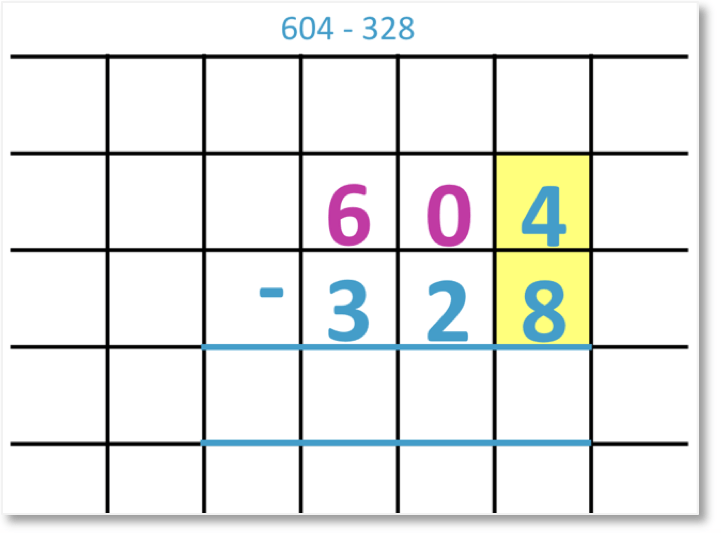# Subtraction with Regrouping

Subtraction with Regrouping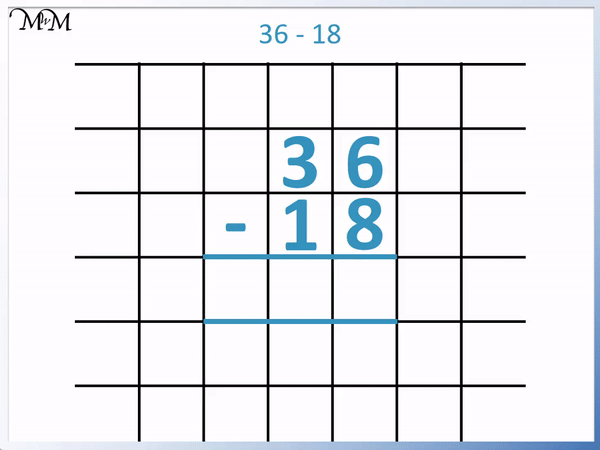• We write the digits in the larger number above the digits in the same place value columns of the smaller number.
• We subtract the digits from right to left.
• We cannot take 8 from 6, so we need to regroup.
• We borrow a ten from the 3 tens in 36.
• 3 tens is reduced to 2 tens and this borrowed ten is moved across to join the 6.
• 6 becomes 16.
• We now subtract 8 from 16. 16 – 8 = 8.
• Now we subtract the tens digits so 2 – 1 = 1.
• 36 – 18 = 18.
• We need to regroup whenever we need to take away a larger digit from a smaller digit.

We regroup when we need to subtract a larger digit from a smaller digit.

We borrow ten from the next place value column along.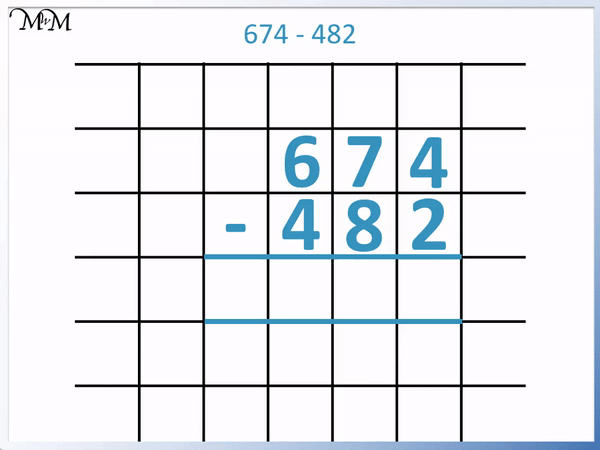• We write the digits in the larger number above the digits in the same place value columns of the other number.
• We subtract from right to left.
• In the ones column, 4 – 2 = 2.
• In the tens column, 8 is larger than 7, so we need to regroup.
• We borrow ten from the next place value column along.
• The 6 is reduced to 5 and we add 10 to 7 to make 17.
• Now we can subtract 8 from 17. 17 – 8 = 9.
• In the hundreds column, 5 – 4 = 1.
• 674 – 482 = 192.Supporting Lessons

#### Subtraction with Borrowing Twice#### Subtraction with Borrowing Twice Worksheets and Answers

Download our printable workbooks for further practice of column subtraction with Borrowing / Regrouping!

# Subtraction with Regrouping

## How to Subtract Numbers using Regrouping

1. Write the largest number first and the number being subtracted below it, lining up the digits.
2. Working from right to left, subtract each digit on the bottom from the digit above it.
3. If any digit on the bottom is larger than the digit above, then regrouping is needed.
4. Subtract 1 from digit that is directly to the left of the digit in the top number.
5. Add 10 to the digit of the top number.
6. Now subtract the bottom number from the top number, writing the answer between the answer lines.

For example, we will look at 592 – 226.

The largest number is 592 and it is written first. The smaller number of 226 is written directly below, with the digits in each place value column lined up.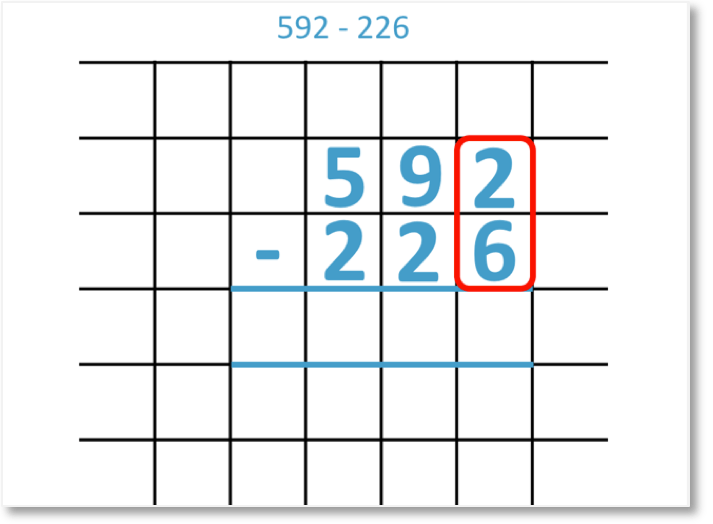We subtract the digits below from the digits above from right to left.

We can see that 6 is larger than 2 and so, regrouping is needed.The digit that is too small is the 2. To regroup (or borrow), we subtract 1 from the digit to the left of this number and add 10 to this number.

We subtract 1 from the 9 tens to leave 8 tens.

We add 10 to the 2 to make 12.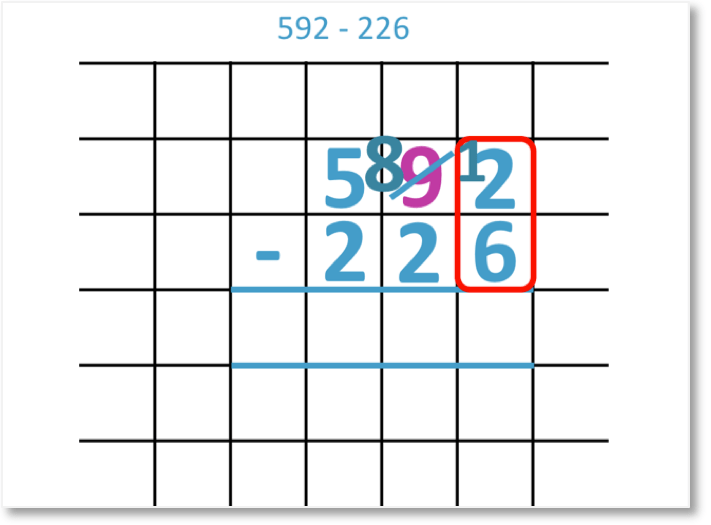We can now subtract 6 from 12. 12 – 6 = 6 and so, we write 6 below.We now subtract the tens column digits.

8 – 2 = 6 and so, we write 6 below.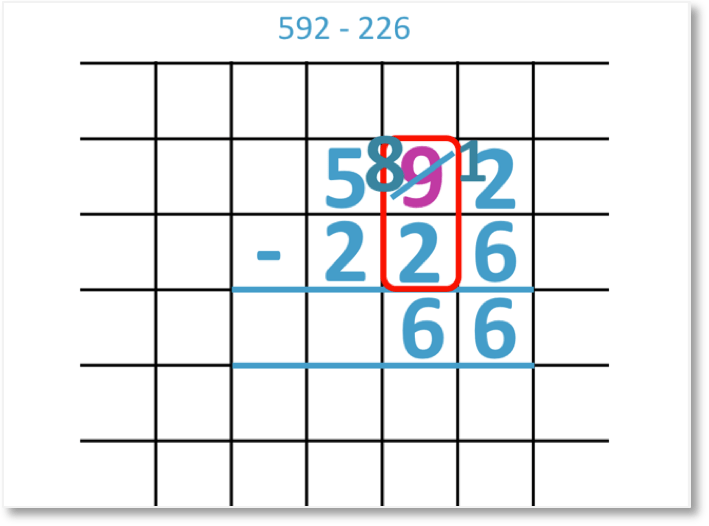We finally subtract the hundreds column digits.

5 – 2 = 3 and so, we write 3 below.592 – 226 = 366. The full subtraction process involving regrouping can be seen below.## What is Subtraction with Regrouping?

Subtraction with regrouping is the process of exchanging one ten with ten ones between place value columns so that a number can be subtracted. This process is commonly known as borrowing.

Regrouping is used in subtraction when the digit being subtracted is larger than the digit you are subtracting from.

For example, in the subtraction of 36 – 18, regrouping is needed because the digit 8 in the ones column is larger than the digit 6.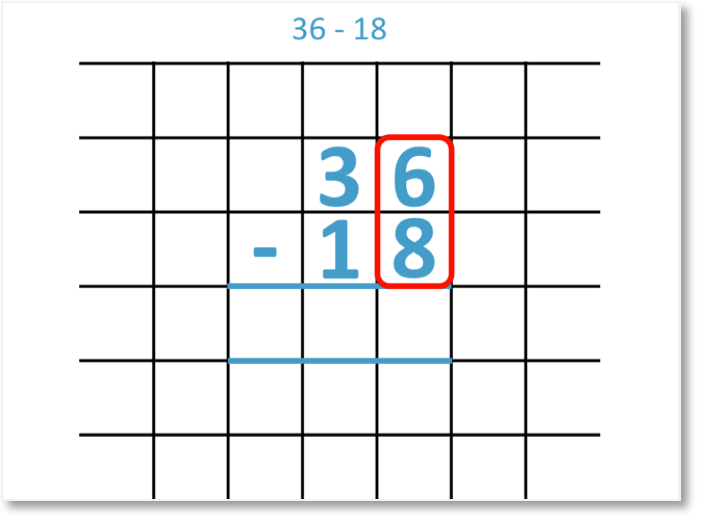Regrouping is part of the column subtraction method shown above, where the number being subtracted is written below the number we are subtracting from.

In column subtraction, we always write each digit in one number directly in line with the digit in the other number that is in the same place value column. When teaching column subtraction, it is helpful to use square grid paper and write each digit in its own box. This helps to keep the digits in the same place value columns in line.

The subtraction is carried out digit by digit, from right to left. Since we cannot subtract 8 from 6, we need to use regrouping.We look at the 3 tens in the tens column.

We regroup by subtracting one from this ten and increasing the units column by ten.

The 3 tens decrease to become 2 tens and because we did this, we add 10 to 6 to make it become 16.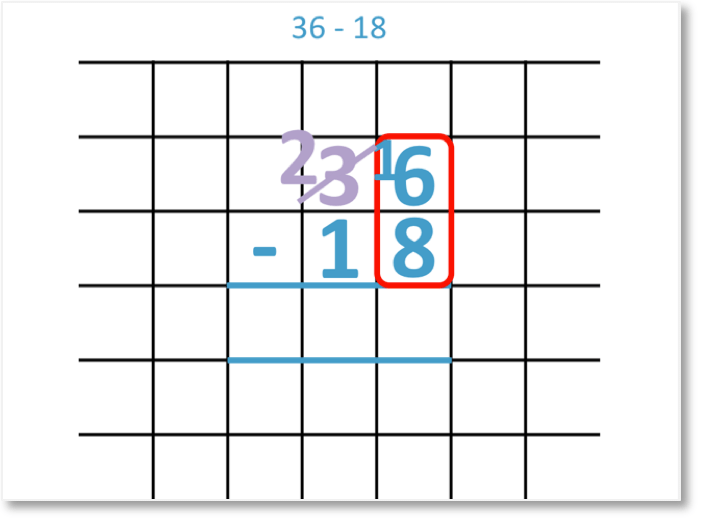We have borrowed ten from the tens column and moved it to the units column. Subtraction by regrouping is often referred to as subtraction by borrowing.

Now the regrouping has been done, we are able to do the subtraction in the units column.

16 – 8 = 8 and so, we write 8 in the answer lines below the subtraction.Now we subtract from the tens column.

We simply have 2 – 1 = 1 and so, we write a 1 between the answer lines below the subtraction.In the column subtraction method we read our answer from the digits between the lines at the bottom.

36 – 18 – 18

Here is the full column subtraction process with the regrouping method shown.## Teaching Subtraction with Regrouping

When teaching subtraction it is helpful to explain regrouping using counters or base ten blocks.

Each number in the subtraction of 36 – 18 can be shown below using counters.

36 is made up of 3 tens and 6 ones. This is shown below with the purple counters. There are 3 rows of ten in the tens column and 6 individual ones in the ones column.

18 is also shown below in green counters. 18 is made up of 1 ten and 8 ones. There is 1 row of ten in the tens column and 8 ones in the ones column.We teach regrouping by trying to subtract the 8 counters from the 6 in the units column.

We do not have enough counters. 8 is 2 larger than 6.

We need to borrow some counters from the tens column. The counters in the tens column only come in sets of ten and so, we move an entire row of ten over from the tens column to the units column.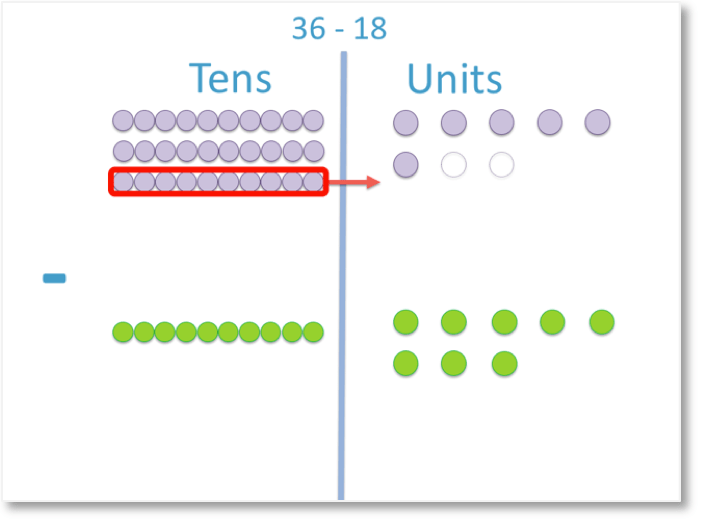We now only have 2 rows of ten purple counters remaining in the tens column but there are 16 individual purple counters in the ones column.This process is called regrouping. We have exchanged one ten for ten ones between the place value columns.

We can now subtract the 8 from the 16.

When teaching subtraction with counters, we can pair up the counters we have with the counters we are subtracting. Every time they pair up we can remove them.We have 8 counters remaining in the units column.We can now subtract the tens column in the same way.

Each time a green counter pairs up with a purple counter it can be subtracted.Once the counters have been removed, we can count how many are left in each place value column.We have 1 group of ten in the tens column and 8 ones in the units column.

36 – 18 = 18 and the full regrouping process is shown taught with counters below.When teaching column subtraction, it can be helpful to show the regrouping process with counters or base ten blocks. It can be helpful to allow the child to play with the counters and try different subtractions until they feel comfortable with the process.

However, it is important to relate this back to the written method and encourage fluency with the written column subtraction procedure.

## 2-Digit Subtraction with Regrouping

When subtracting 2-digit numbers, regrouping is used to exchange 1 group of ten with ten ones.

The digit in the tens column is reduced by 1 and the digit in the ones column is increased by ten.

Here is the example of 92 – 27.

We set the subtraction out as a column subtraction, with the larger number on top and the smaller number directly below. The digits in the smaller number are written directly below the digits in the same place value columns of the larger number.

We subtract the digits from right to left, starting with the units column.Since 7 is larger than 2, we need to borrow from the tens column.

This means that we borrow a ten from 90 and add it to the 2.

90 becomes 80 and so, 2 becomes 12.

We can now subtract the digits in both columns.

12 – 7 = 5 and

8 – 2 = 6

92 – 27 = 65.

Here is another example of subtracting 2-digit numbers using regrouping.

We have 64 – 36. Looking at the digits in the units column, 6 is larger than 4 and so, regrouping will be used.

We borrow ten from the tens column.

This means that in the number 64, 60 becomes 50 and the 4 becomes 14.We can now do the subtraction.

14 – 6 = 8 and

5 – 3 = 2

64 – 36 = 28.

## 3-Digit Subtraction with Regrouping

When subtracting a 3-digit number, we may need to regroup one ten as ten ones or we may regroup one hundred as ten tens.

Here is an example of subtracting a 3-digit number using regrouping. We have 674 – 482.

We write the number being subtracted below the larger number, lining up the digits in each place value column.

We start by subtracting the digits in the ones column on the right.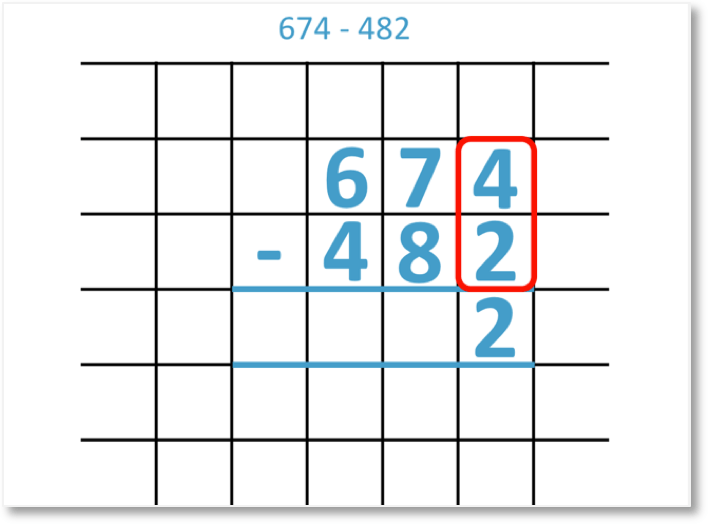4 – 2 = 2

In the tens column, 8 is larger than 7, so we will need to regroup one hundred as ten tens.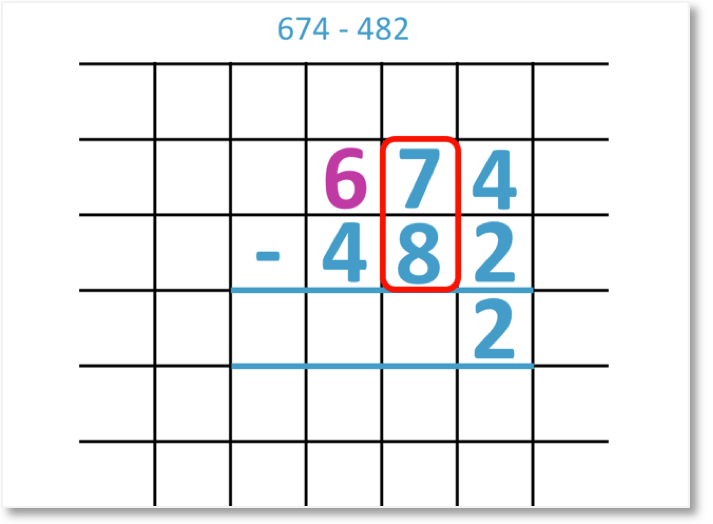We subtract one from the digit of 6 to make 5 hundreds.

We add ten to the digit of 7 to make 17 tens.

One hundred was exchanged for ten tens.We can now subtract the digits in the tens column.

17 = 8 = 9 and so, we write 9 in the answer space below.We now subtract the digits in the hundreds column.

5 – 4 = 1 and so, we write 1 below in the answer space.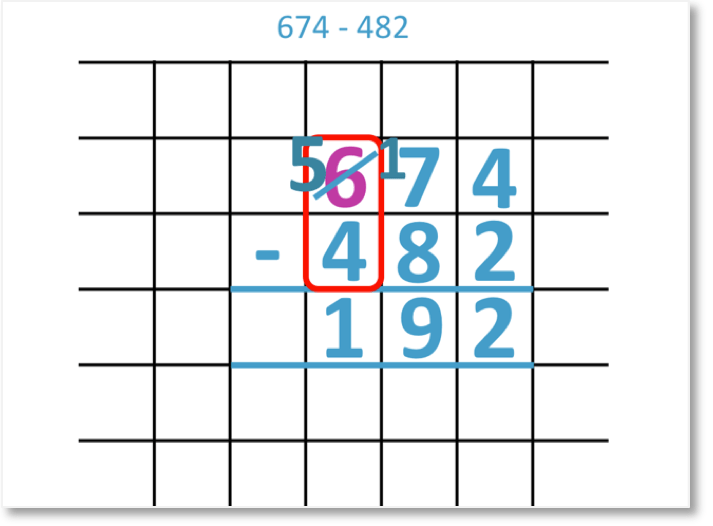674 – 482 = 192. The full 3-digit subtraction process including the borrowing steps is shown below.Here is another example of subtracting 3-digit numbers involving borrowing.

We have 835 – 561.

We first subtract the ones column digits like usual.

5 – 1 = 4

Next we look at the tens column digits and see that 6 is larger than 3. We need to regroup from the hundreds column.We subtract 1 from the hundreds column and increase the tens column by 10.

8 becomes 7 and 3 becomes 13.

We can now subtract the tens column digits.

13 – 6 = 7 and so, we write 7 below.

We can now subtract the hundreds column digits.

7 – 5 = 2 and so, we write 2 below.

835 – 561 = 274

## Subtraction with Regrouping Twice

Subtracting 3-digit numbers may sometimes result in regrouping twice. Regrouping twice occurs if both the digits in the tens and ones columns of the smaller number are larger than the tens and ones digits of the larger number.

For example in the 3-digit subtraction of 346 – 178, we can see that both the digits in the tens and ones columns of the smaller number are larger than the digits in the tens and ones columns of the larger number.

We start by looking at the units column where 8 is larger than 6.We will need to regroup from the tens column.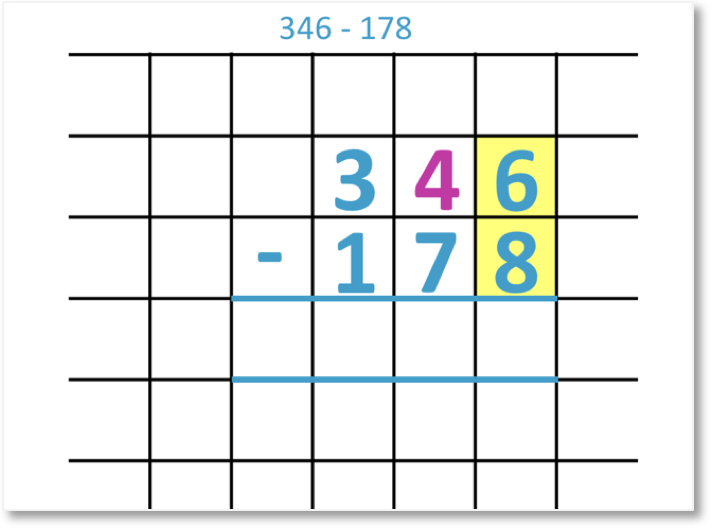We subtract one from the tens column so that 4 becomes 3.

We add ten to the ones column so that 6 becomes 16.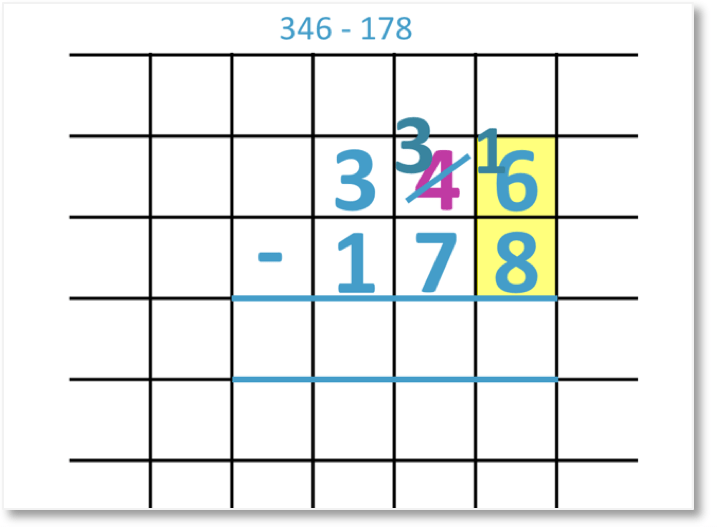Now we can subtract the units column digits.

16 – 8 = 8We now look at the tens column but 7 is larger than 3. We need to regroup again.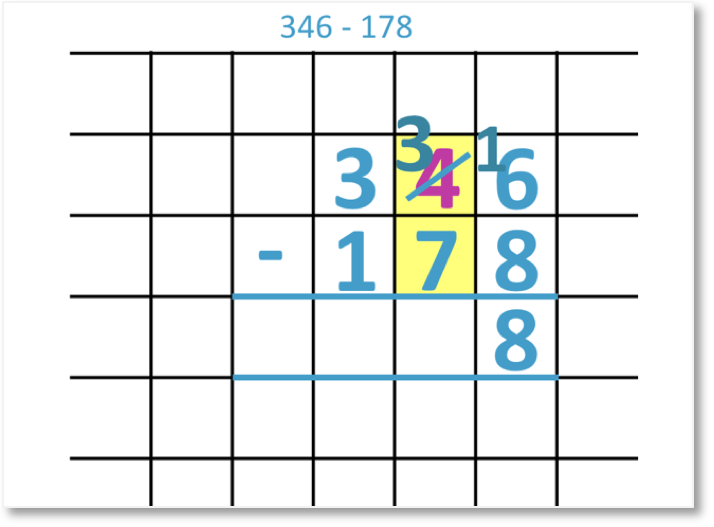We will regroup one hundred as 10 tens.

We will subtract 1 from the hundreds column and increase the tens column by 10.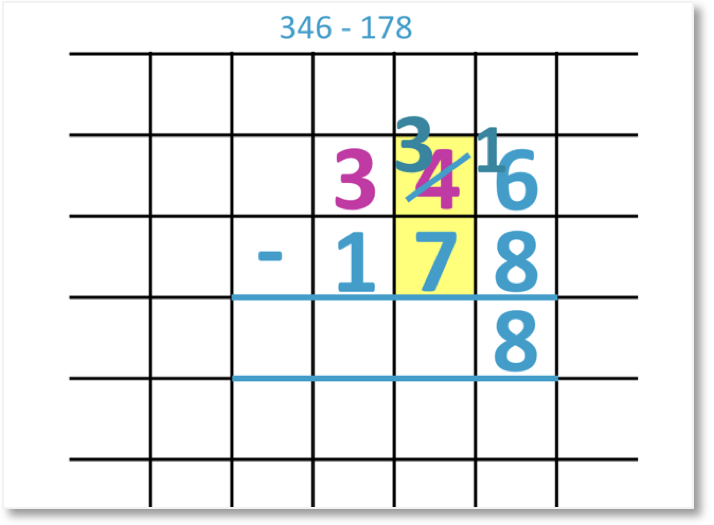The 3 becomes 2 in the hundreds column and in the tens column, we add 10 to 3 to make 13.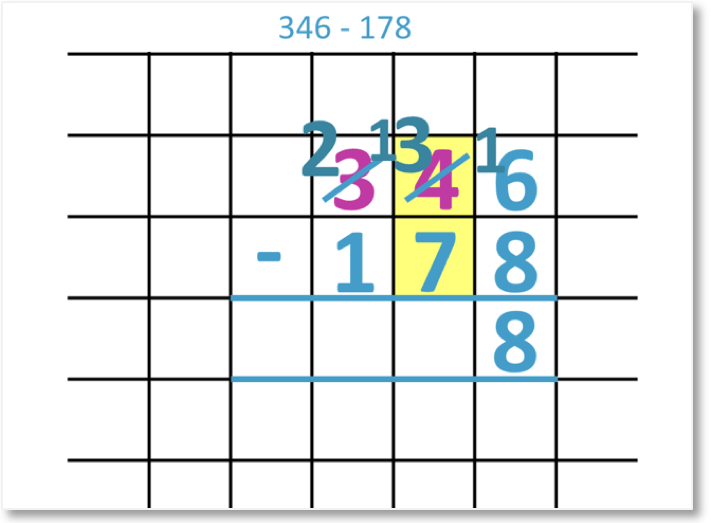We can now subtract the tens column digits so that 13 – 7 = 6. We write 6 below in the answer space.We can finally subtract the hundreds column digits.

2 – 1 = 1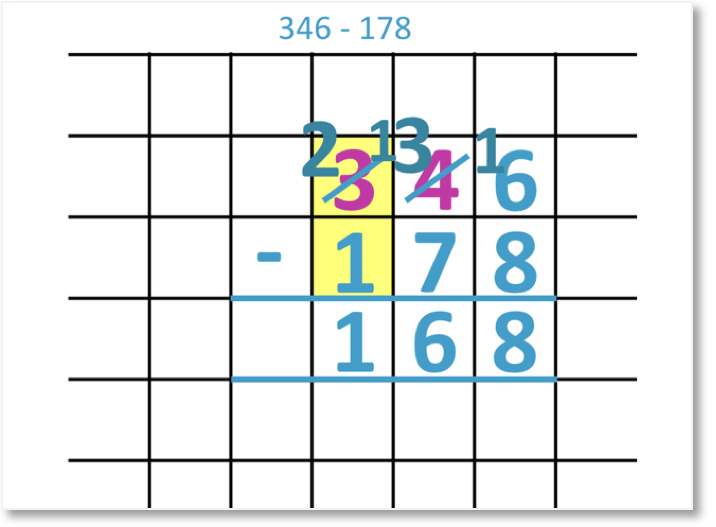We can see that 346 – 178 = 168.

The full process of regrouping twice is shown below.Here is another example of subtraction with regrouping twice.

We have 651 – 254.

We can see that in this example, the ones column of the smaller number is larger than the ones column of the larger number. The tens digits in both numbers are both 5.

Since 4 is larger than 1, we first regroup from the tens column.

In the tens column, 5 is reduced to a 4 and in the ones column, 1 is increased to 11.

We can subtract 4 from 11 to get 7 in the ones column.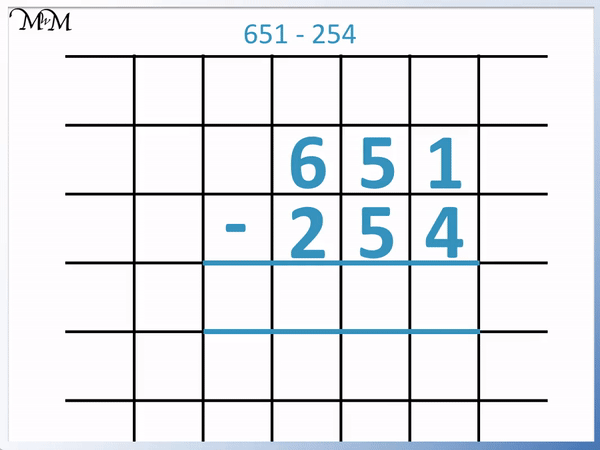Now we have reduced the tens column digit, we can no longer perform the subtraction in the tens column. 5 in the bottom number is larger than the 4 in the top number.

We need to regroup from the hundreds column.

6 is reduced to 5 in the hundreds column and the 4 is increased to 14 in the tens column.

We can now complete the subtraction.

In the tens column, 14 – 5 = 9 and in the hundreds column, 5 – 2 = 3.

651 – 254 = 397

Here is another example of subtraction with borrowing twice. We have 432 – 386.

We can see that the tens and ones digits in the smaller number are larger than the tens and ones digits of the larger number.

We regroup from the tens column so that the 3 becomes a 2 and in the ones column, the 2 becomes a 12.

We can subtract the ones column digits so that 12 – 6 = 6.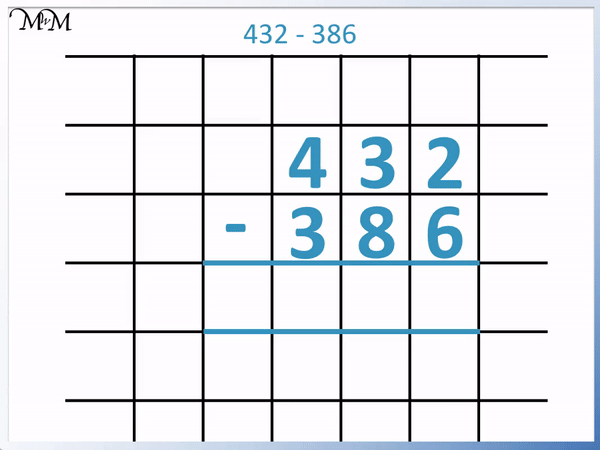We cannot subtract the tens digits without regrouping because 8 is larger than 2.

We regroup from the hundreds column so that 4 becomes 3 and in the tens column, 2 becomes 12.

We can now subtract the tens column digits so that 12 – 8 = 4.

In the hundreds column, 3 – 3 = 0.

We do not have any hundreds remaining so we do not write them.

432 – 386 = 46Now try our lesson on Subtraction Borrowing from Zero where we learn how to borrow from zero in a subtraction.